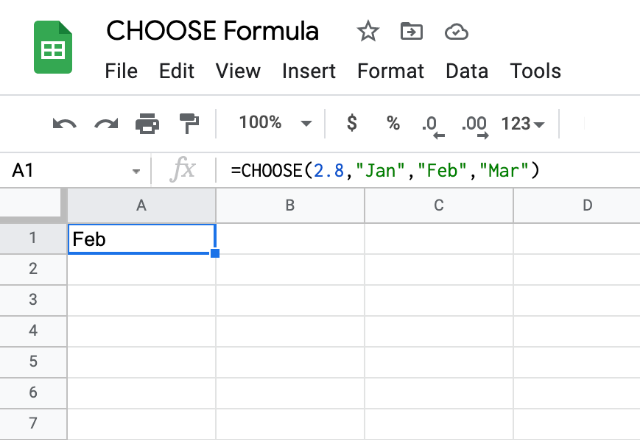# How to use the CHOOSE formula in Google SheetsWe may already be aware of the usability of the nested IF statements and SWITCHformulas in Google Sheets. Going by the experience, we can conclude that the SWITCH formula is less complicated than that of the nested IF statement. On the similar lines, wouldn’t it be nice if instead of specifying the case every time, we just specify an index of sorts, and we get the corresponding value? Well, just to help us with that purpose, the Google Sheets application hosts the CHOOSE formula.

### Syntax

CHOOSE(index, choice1, [choice2, …])

• index – this specifies the choice number. If it is 1, the CHOOSE formula returns the choice1. Similarly, if it is 3, we get choice3 in return. Please note that the Google Sheets allows up to 30 index values, starting from 1.
• choice1 – the formula returns this when the index value is 1. It can be a simple text, number, reference to another cell, or even a full-fledged formula.
• choice2 – additional and optional parameters similar to that of choice1.

### Usage: CHOOSE Formula

Let us start with the basic example that returns the weekday based on the index number. In the snapshot below, you can see the various combinations of the formula that we tried.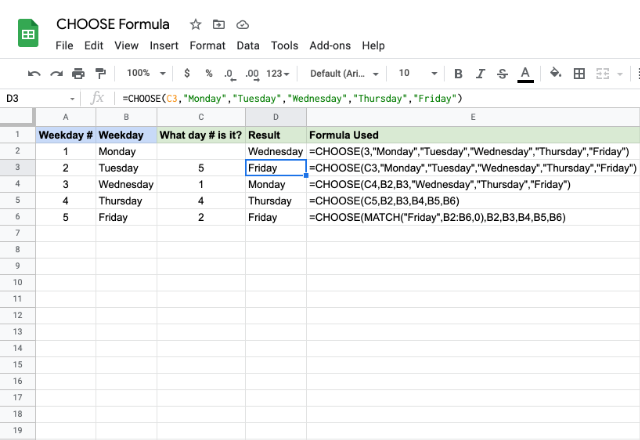Like we mentioned above, the range of the index values can only start from 1 and can’t exceed the total number of choices within the formula. What happens if we try to force that? It coughs up errors of course! Let us try that firsthand. Below is the snapshot that demonstrates the outcome of using the index value 0.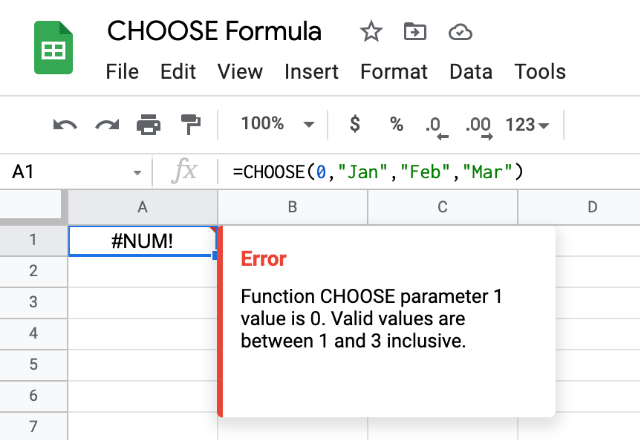Does the behavior change when we exceed the number of choices (in this case 3)? As it turns out, not really.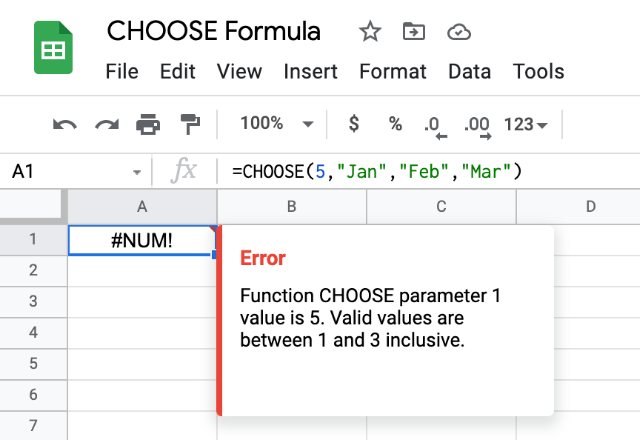Similar is the case when the index number is either a negative number.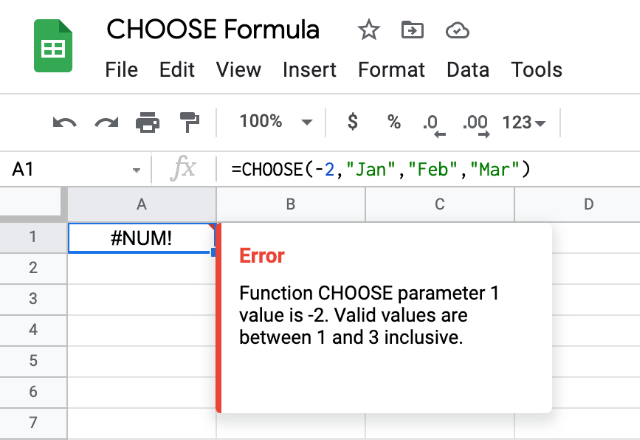What happens if the index is a decimal number? Surprisingly, it doesn’t error out this time. The CHOOSE formula considers the value on the left of the decimal point (in this case 2) and evaluates the formula accordingly.